# In-Context Pretraining Language Modeling Beyond Document Boundaries

This is my reading note for In-Context Pretraining: Language Modeling Beyond Document Boundaries. This paper proposes to group relevant instead of random documents in each batch to improve Long text learning. The relevant docs are found by performs a traveling salesmen problem in a graph of documents. The edges of two documents define whether the two documents are in the top k nearest neighbors.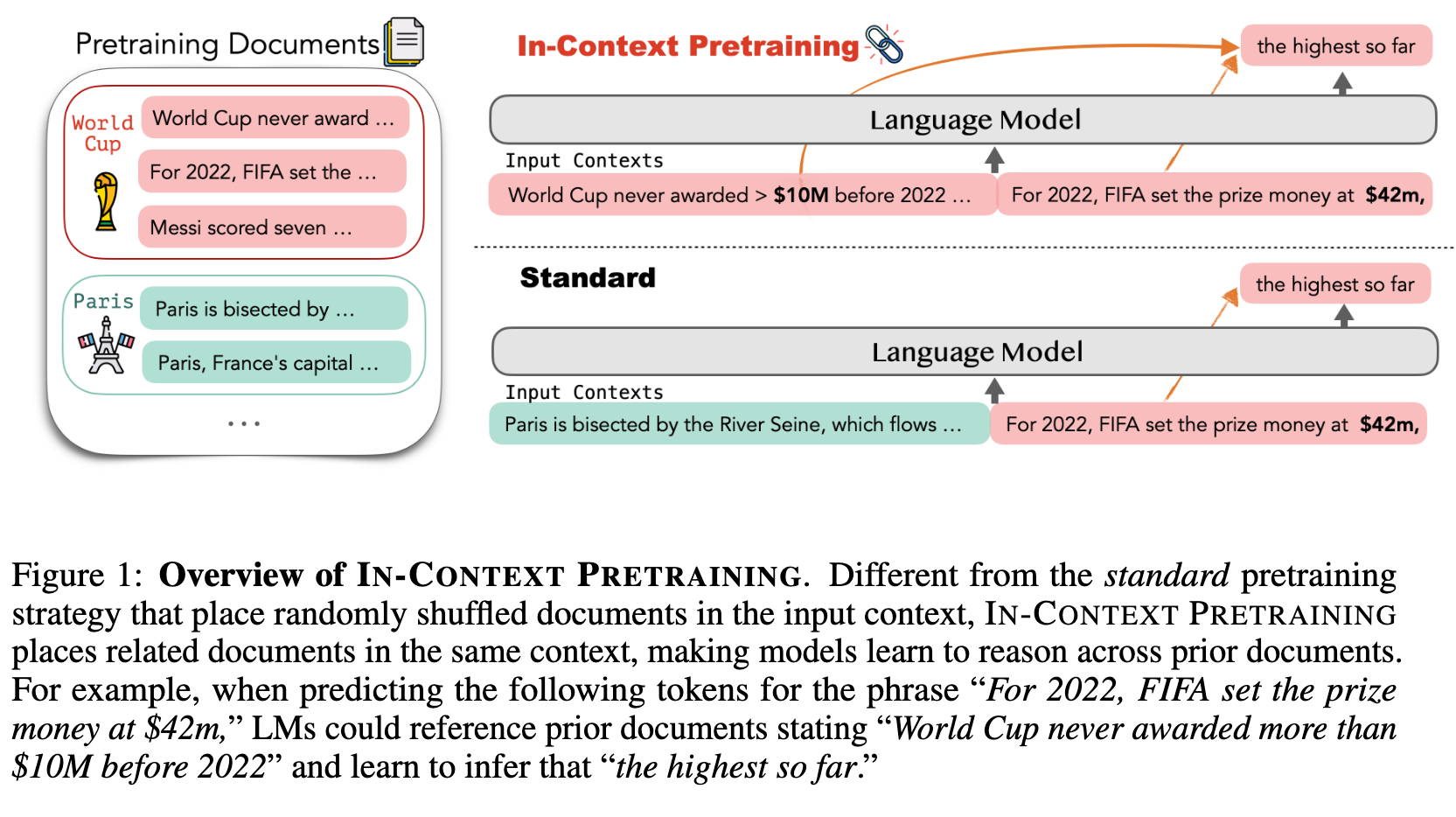# Introduction

Existing pretraining pipelines train LMs by concatenating random sets of short documents to create input contexts but the prior documents provide no signal for predicting the next document. We instead present IN-CONTEXT PRETRAINING, a new approach where language models are pretrained on a sequence of related documents, thereby explicitly encouraging them to read and reason across document boundaries. (p. 1)

To do this, we introduce approximate algorithms for finding related documents with efficient nearest neighbor search and constructing coherent input contexts with a graph traversal algorithm. (p. 1)

We use a retrieval model paired with an efficient search index to build a document graph that pairs each document with its nearest-neighbors based on its semantic similarity in the embeddings space. We also formulate document sorting as a travelling salesman problem, for which we develop an effective algorithm that maximizes similarity of documents to their context while also ensures that each document is included only once. (p. 2)

# RELATED WORK

Several studies explore pretraining language models on a small-scale using related documents. For example, Yasunaga et al. (2022) incorporate Wikipedia documents with hyperlinks or citations into the input context and pretrain a masked LM. Caciularu et al. (2021) gather related documents using a human-curated multi-document news summarization dataset (11 million tokens) and continue to pretrain a masked LM. Lewis et al. (2020) place documents from the same date in the input context and pretrain LMs to summarize articles. (p. 9)

## Multitask finetuning for in-context and instruction learning

Finetuning language models on a collection of downstream tasks to improve the instruction learning and in-context learning abilities of LMs has been investigated in several papers. As discussed by Min et al. (2022); Chen et al. (2022); Ivison et al. (2023); Wang et al. (2022; 2023b), a prevailing technique concatenates instructions, training samples from human-annotated downstream datasets into single text sequences, upon which the LM is subsequently finetuned. Following this line of work, Gu et al. (2023) create intrinsic downstream datasets by developing a task-specific retriever for each task. These retrievers are then used to retrieve demonstration examples from the pretraining corpus. (p. 9)

## Training long-context language models

Press et al. (2022); Chen et al. (2023); kaiokendev (2023) make modifications to position encoding and finetune LMs on randomly concatenated short documents and subsampled long documents from pretraining data. However, as highlighted by de Vries (2023), long sequence documents are notably rare in the pretraining data. For example, less than 5% of documents in CommonCrawl have longer than 2k tokens. (p. 9)

# IN-CONTEXT PRETRAINING

Specifically, for each document d_i ∈ D, a dense retrieval model is used to retrieve the top-k most similar documents, represented as N(d_i). The retrieval model uses approximate nearest neighbours search for efficient pairwise similarity comparison between any two documents, making it scalable for finding related documents in web-scale pretraining corpora. (p. 3)

Our retrieval process employs the contriever model (Izacard et al., 2022). This model maps each document d_i ∈ D to an embedding E(d_i) by taking the mean pooling of the last hidden representation over the tokens in d_i. The cosine similarity is then used to determine the similarity between any two documents (p. 3)

The retrieval model uses approximate nearest neighbour search, product quantization (Jégou et al., 2011) and an inverted file FAISS index (Johnson et al., 2019) to conduct efficient pairwise similarity search. we found that the pretraining corpus contains many near duplicate documents. Hence, we further leverage the retrieval scores to eliminate near duplicate documents from the pretraining corpus. (p. 3)

## CREATING INPUT CONTEXTS: DOCUMENT GRAPH TRAVERSAL

Formally, we aim to form a set of input contexts C_1 · · · C_m where each context C_i = {d_1, …d_k} ⊂ D and $\union_{i=1}^m C_i=D$. Ideally, documents in C_i are nearest neighbors of each others. (p. 3)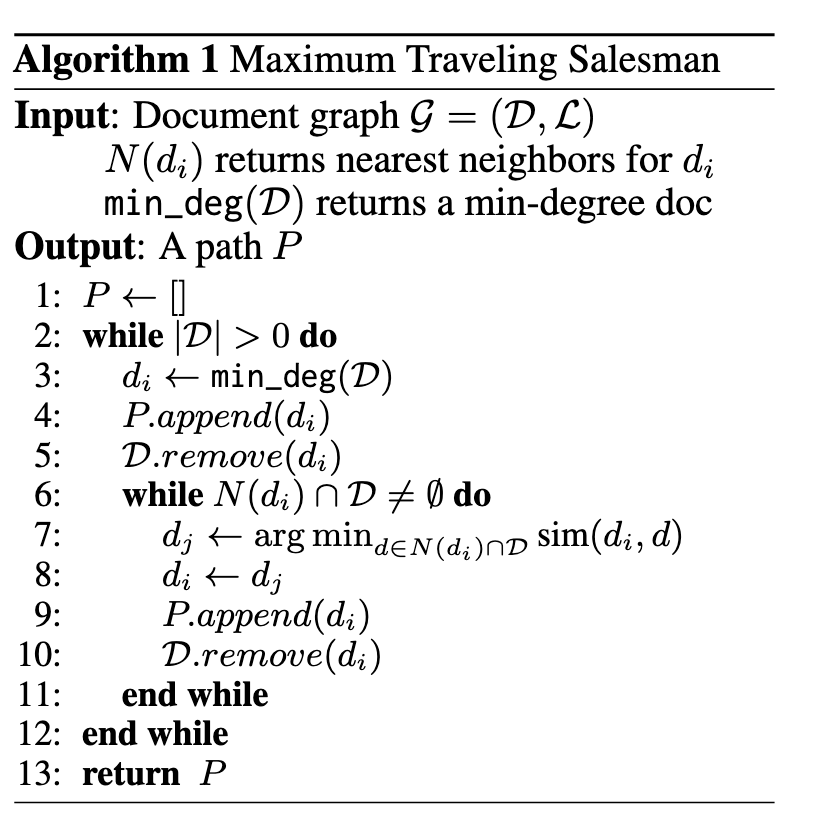A straightforward approach to form C_1 · · · C_m is to directly place each document and its retrieved top_k documents together in the same input context (referred to as kNN), (p. 3)

This kNN approach maintains document similarity within each context but creates the data repeating problem: some documents frequently appear as nearest neighbors of other documents, causing that different input contexts contain overlapping documents, i.e., $\exists i \neq j]mbox{, } C_i \intersect C_j \neq ∅$. The data repeating problem exposes LMs to a less diverse set of documents given a fixed computational budget and could lead to overfitting of popular documents. (p. 3)

an intuitive approach is to find a single path that visits each document once and maximize the chance that related documents are visited sequentially. Then we subsequently segment the path into multiple input contexts. We formulate it as the maximum traveling salesman problem (Flood, 1956) that aims to find the maximum weight path that traverses all nodes exactly once. (p. 4)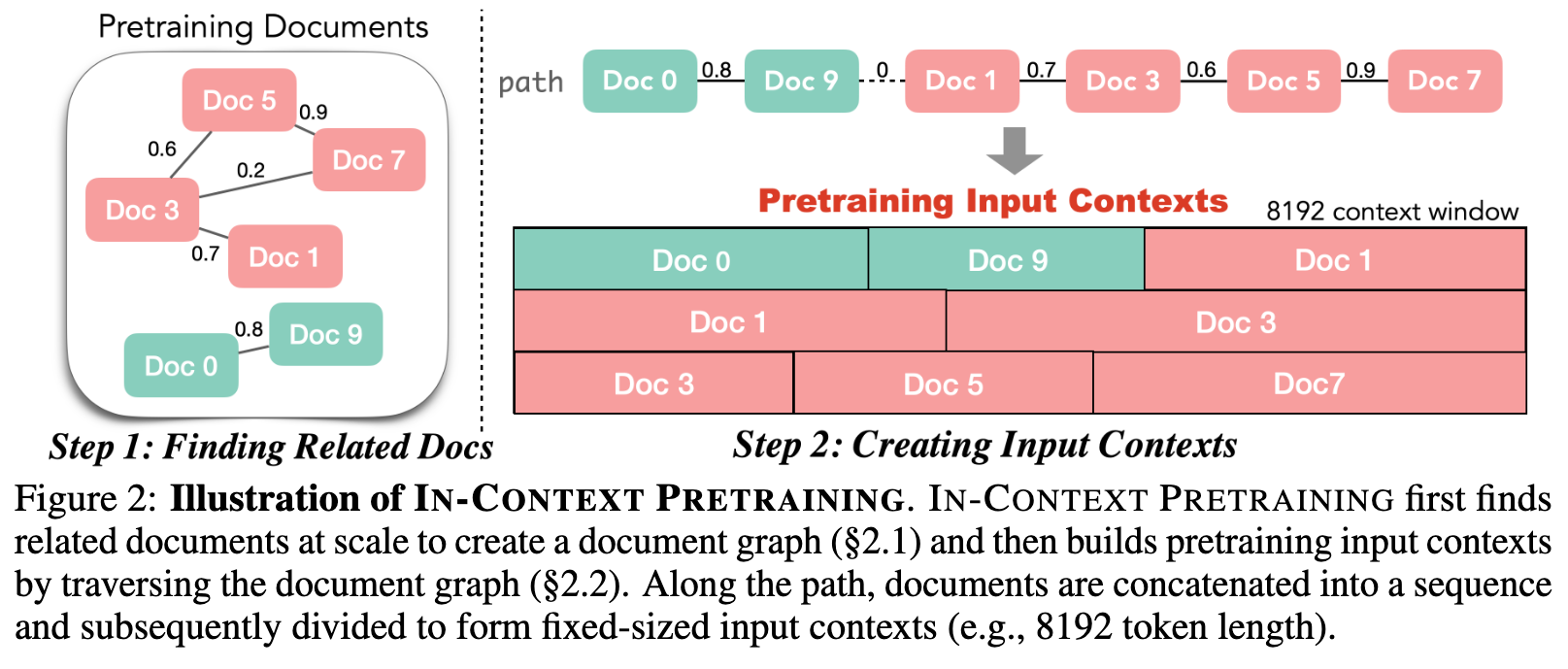Solving large traveling salesman problems exactly is NP hard, but greedy algorithms are known to provide an efficient approximate solution. Our algorithm starts by selecting a yet-to-be-visited document with the minimum degree as the starting node (Doc 0). The algorithm then progressively extends the current path by navigating to its unvisited neighboring document with highest weight (Doc 9), adding the document node to the path. In this case, we extend the graph with an edge of weight 0 to a random unvisited minimum degree document (Doc 1), and continue the above process. The motivation for starting at minimum degree documents is that they are most likely to have all their neighbors visited first, and therefore be connected to dissimilar documents in the final path. (p. 4)

# EXPERIMENTS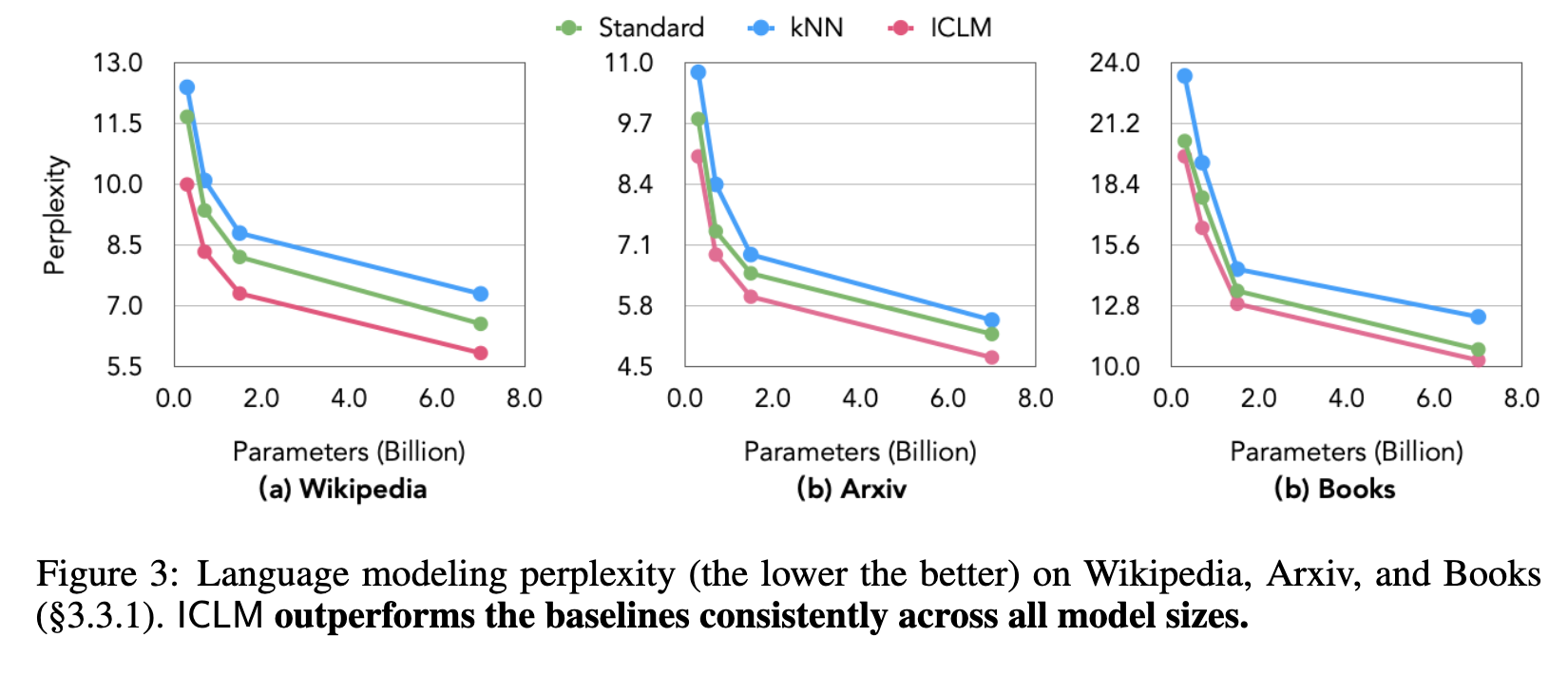Model Details. We take the model architecture from LLaMA (Touvron et al., 2023a) and train models across various sizes: 0.3, 0.7, 1.5, and 7.0 billion parameters, all with an 8192-length context window. Following LLaMA, we employ the AdamW optimizer (Loshchilov & Hutter, 2018) with parameters β1 = 0.9 and β2 = 0.95, and a cosine learning rate schedule. The 7B model is pretrained using 128 A100 GPUs across 16 nodes with a batch size of 4 million tokens. It takes 9 days to train the 7B model on our pretraining dataset. Due to the long context window of our models, we use flash attention (Dao et al., 2022) to reduce memory consumption during pretraining. (p. 5)

To perform the retrieval over our pretraining datasets, we construct FAISS big batch search that is designed for conducting efficient similarity search with big batches of vectors (typically 50M–100M vectors per batch). We split the data in batches of 50M embeddings, the search step is conducted in each batch before merging the results using 8 GPUs per batch. The total search time is 6 hours with average search time per batch is 4,738s. (p. 5)

First, kNN does not improve over the standard LM, likely due to the overfitting problem as discussed in §2.2. ICLM, in contrast, outperforms both the standard LM and kNN on all three datasets, even when the evaluation documents are not sorted. The gains are consistent or larger as the size of the model scales (p. 5)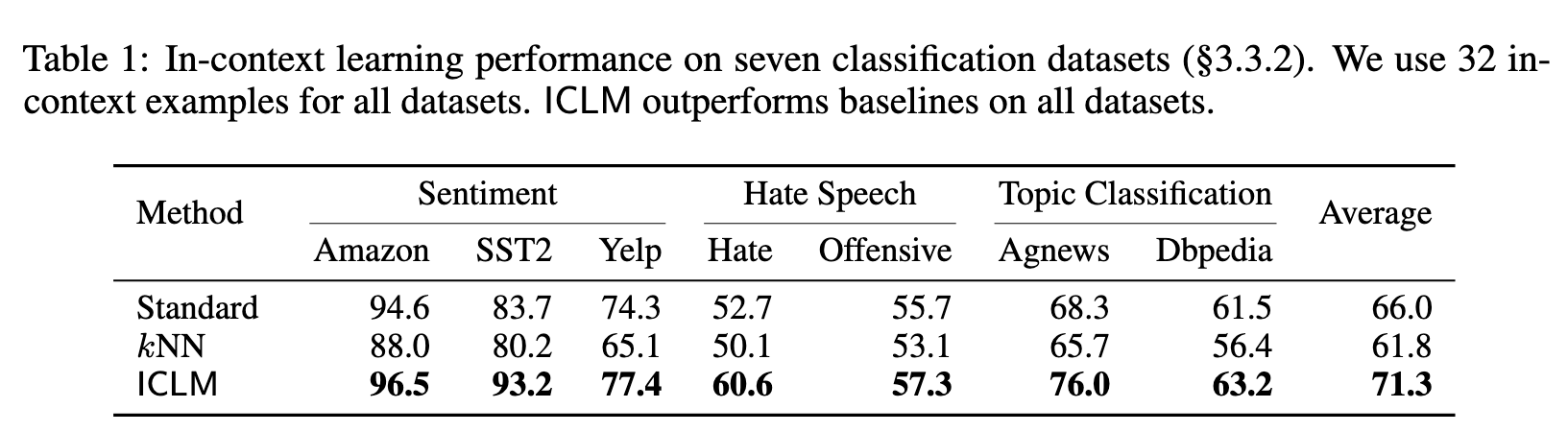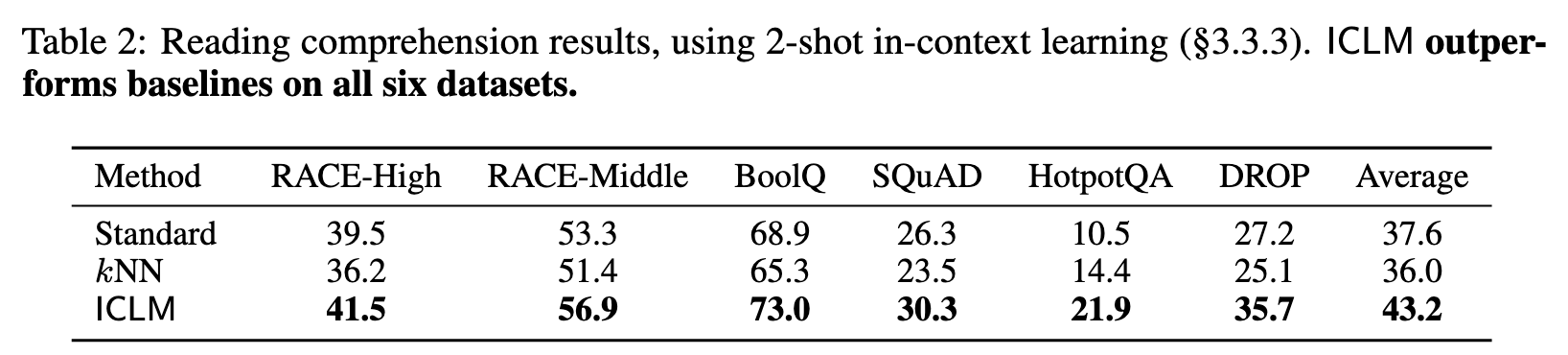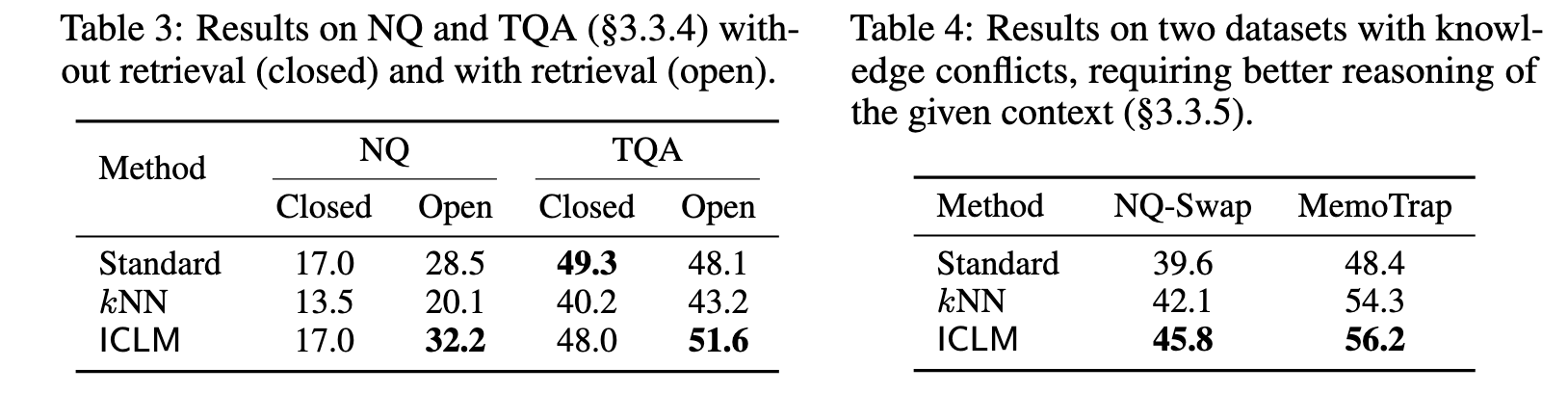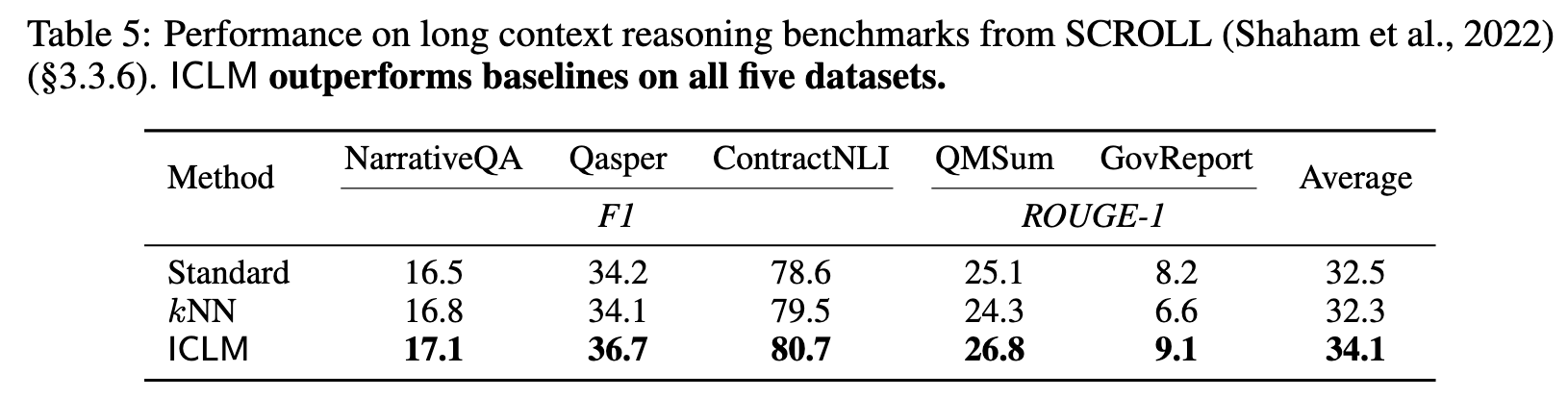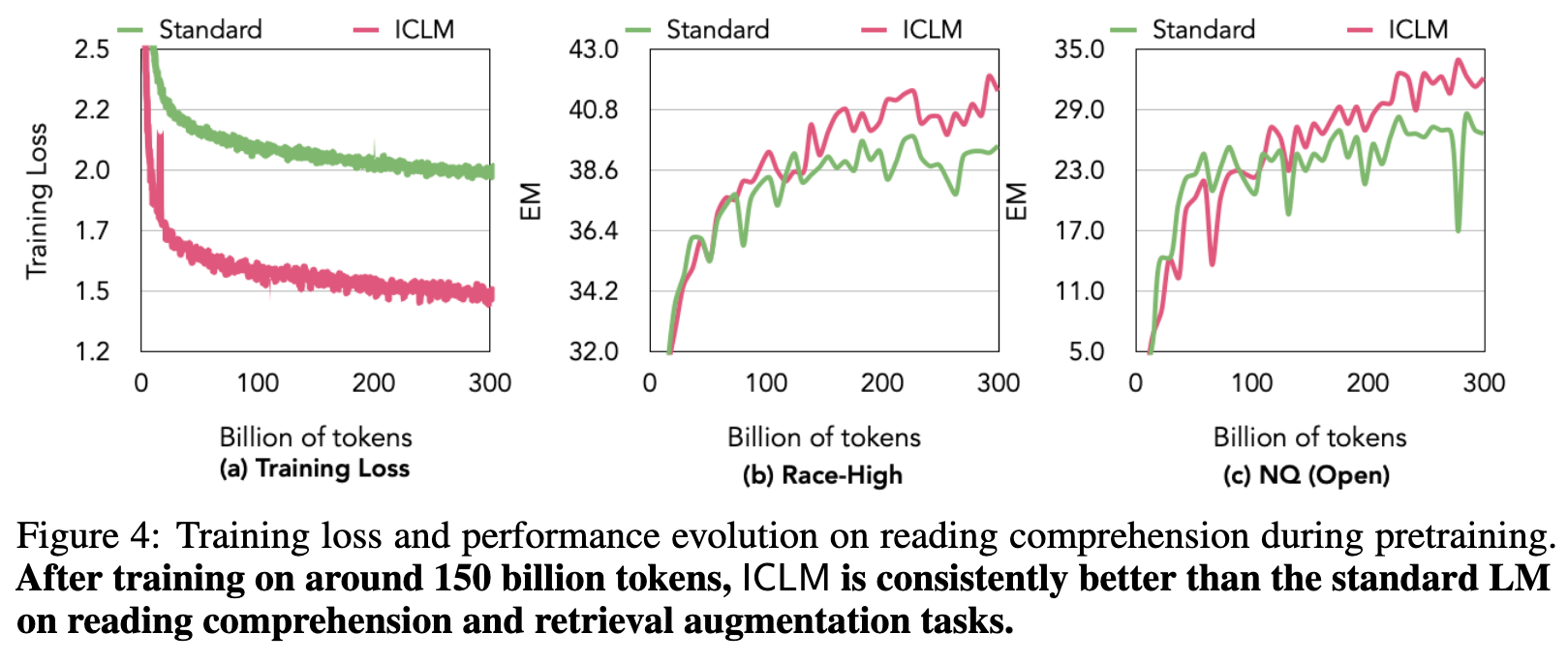# ABLATION STUDY ON IN-CONTEXT PRETRAINING DESIGN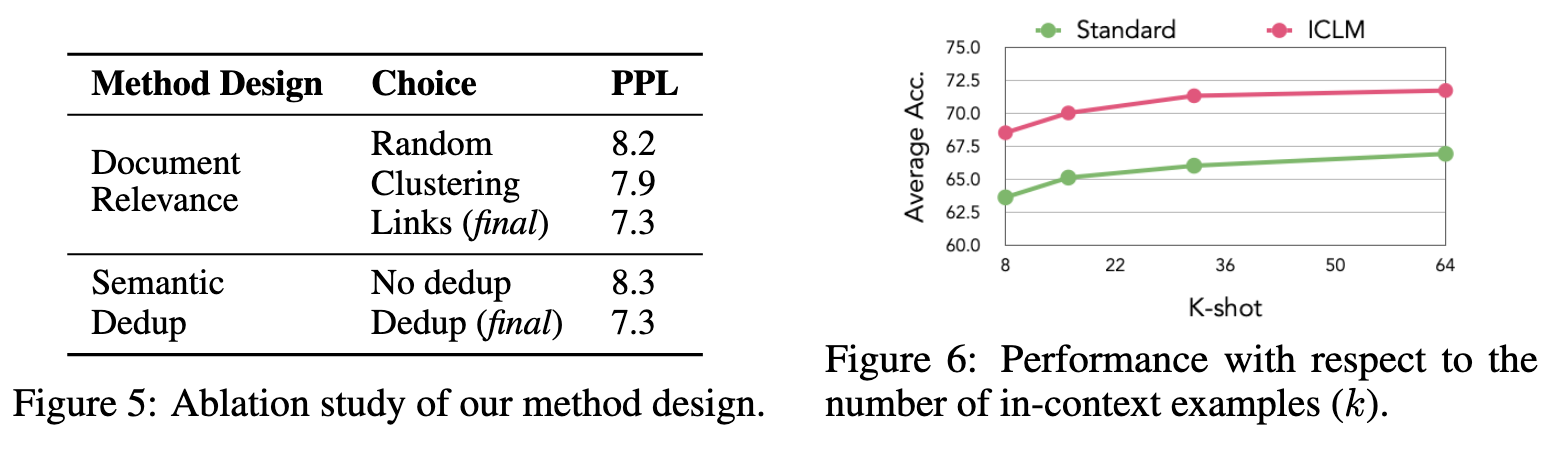## Document relevance

A key design of IN-CONTEXT PRETRAINING is grouping documents by their relevance. We consider three levels of relevance: random (the standard baseline discussed in §3.2), clustering, and our document linking method in IN-CONTEXT PRETRAINING. Clustering follows the method from Abbas et al. (2023) in clustering documents into 11k clusters based on their embeddings and sample documents from each cluster to form the training inputs. The relevance between documents increases from random, clustering to linking. We observe that the perplexity of the language model decreases as the relevance increases. (p. 8)

## Deduplication

We compare perplexity of the models trained with and without the semantic deduplication step. Removing the semantic deduplication step leads to a significant decrease in perplexity. When near duplicate documents are present in the same context, language models might merely copy from the prior document, leading to training instability. (p. 9)

Written on October 18, 2023